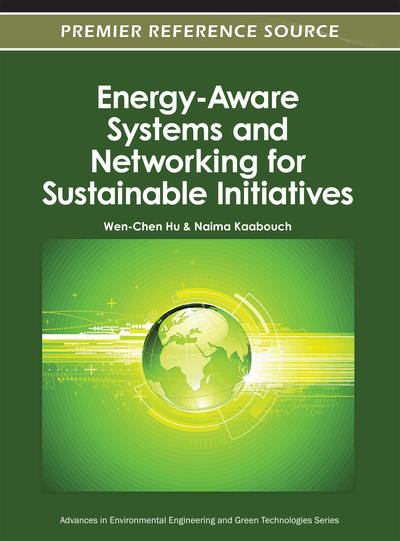Energy Efficient Routing by Switching-Off Network Interfaces

Frédéric Giroire (INRIA, France), Dorian Mazauric (INRIA, France) and Joanna Moulierac (INRIA, France)
DOI: 10.4018/978-1-4666-1842-8.ch010
Available
\$37.50
No Current Special Offers

Abstract

Several studies exhibit that the traffic load of routers only has a small influence on their energy consumption. Hence, the power consumption in networks is strongly related to the number of active network elements, such as interfaces, line cards, base chassis, etc. The goal is to find a routing that minimizes the (weighted) number of active network elements used when routing. In this chapter, the authors consider a simplified architecture where a connection between two routers is represented as a link joining two network interfaces. When a connection is not used, both network interfaces can be turned off. Therefore, in order to reduce power consumption, the goal is to find the routing that minimizes the number of used links while satisfying all the demands. The authors first define formally the problem and model it as an integer linear program. Then, they prove that this problem is not in APX, that is there is no polynomial-time constant-factor approximation algorithm. They propose a heuristic algorithm for this problem and also prove some negative results about basic greedy and probabilistic algorithms. Thus, the authors present a study on specific topologies, such as trees, grids, and complete graphs, that provides bounds and results useful for real topologies. They then exhibit the gain in terms of number of network interfaces (leading to a global reduction of approximately 33 MWh for a medium-sized backbone network) for a set of existing network topologies: the authors see that for almost all topologies more than one third of the network interfaces can be spared for usual ranges of operation. Finally, the authors discuss the impact of energy efficient routing on the stretch factor and on fault tolerance.
Chapter Preview
Top

1. Introduction

The minimization of ICT energy consumption has become a priority with the recent increase of energy cost and the new sensibility of the public, governments and corporations towards energy consumption. ICT alone is responsible of 2% to 10% (depending on the estimations) of the world consumption (Chiaraviglio, Leonardi, & Mellia, 2009).

In this chapter, we are interested in the networking part of this energy consumption, and in particular in the routing. It is estimated that switches, hubs, routers account for 6 TWh per year in the US (Nordman & Christensen, 2011).

Some recent studies (Chabarek, Sommers, Barford, Estan, Tsiang, & Wright, 2008; Mahadevan, Sharma, Banerjee, & Ranganathan, 2009) exhibit that the traffic load of the routers only has a small influence on their energy consumption. Hence, the dominating factor is the number of switched-on network elements: interfaces, platforms, routers, etc. In order to minimize energy, we should try to use as few network elements as possible.

Nevertheless, in most of networks, PoPs or even routers cannot be turned off. As a matter of fact, first, they are the source or destination of demands; second, they can be part of backup routes to protect the network again failures. For this reason, we consider in this chapter a simplified architecture where a connection between two routers is represented by a link joining two network interfaces.

We can spare energy by turning off the two network interfaces which are the extremities of the link. The network is represented by an undirected graph and, in that case, the goal in this simplified architecture is to find a subgraph minimum in number of links to route the demands. The contributions of this chapter are the following:

• We prove that there is no polynomial-time constant-factor approximation algorithm for this problem, even for two demands or if all links have the same capacity, unless P=NP.

• We present heuristics to find close to optimal solutions for general networks. These heuristics are validated by comparison with theoretical bounds for specific instances of the problem. Furthermore, we exhibit negative results about greedy and probabilistic heuristic algorithms.

• We give explicit close formulas or bounds for specific topologies, such as trees, complete graphs, and square grids. They provide limit behaviors and give indications of how the problem behaves for general networks.

• We study the energy gain on a set of topologies of existing backbone networks. We exhibit that at least one third of the network interfaces can be spared for usual range of demands.

• Finally, we discuss the impact of energy-efficient routing on route length and fault tolerance.

Complete Chapter List

Search this Book:
Reset